Functions And Surfaces

(requires JavaScript)

1. Match functions with their graphs, explain.

1. $f\left(x,y\right)=\left|x\right|+\left|y\right|$
2. $f\left(x,y\right)=\left|xy\right|$
3. $f\left(x,y\right)=\frac{1}{1+{x}^{2}+{y}^{2}}$
4. $f\left(x,y\right)={\left({x}^{2}-{y}^{2}\right)}^{2}$
5. $f\left(x,y\right)={\left(x-y\right)}^{2}$
6. $f\left(x,y\right)=\mathrm{sin}\left(\left|x\right|+\left|y\right|\right)$
1.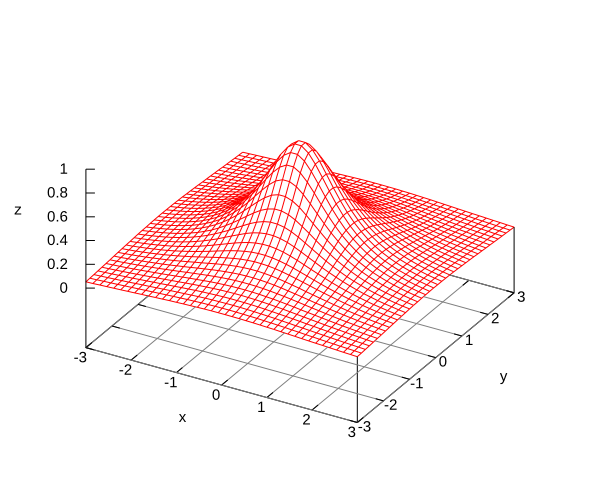2.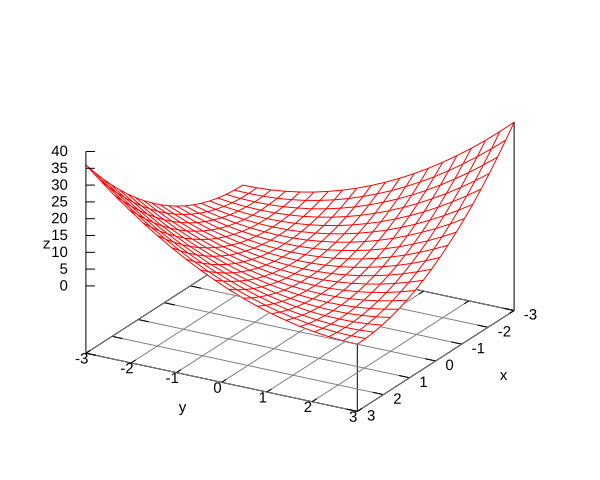3.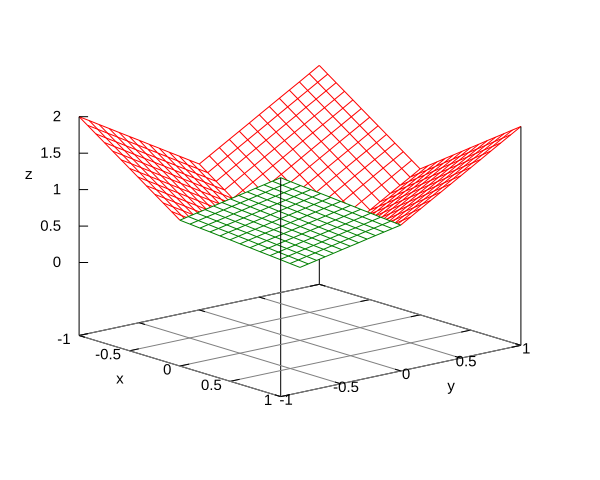4.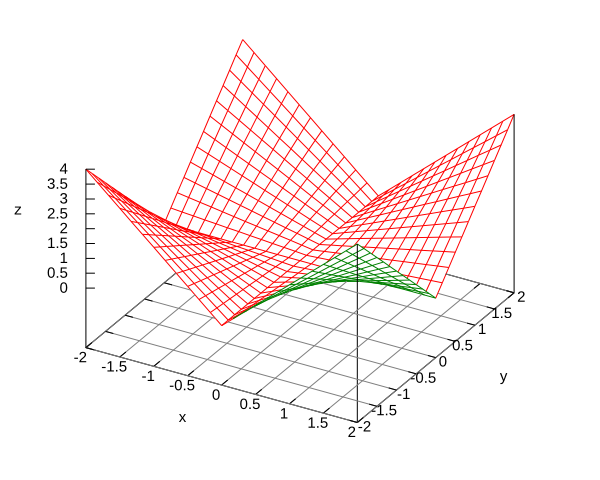5.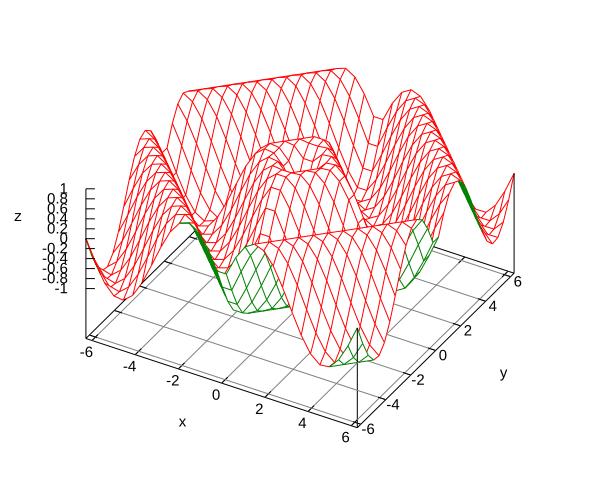6.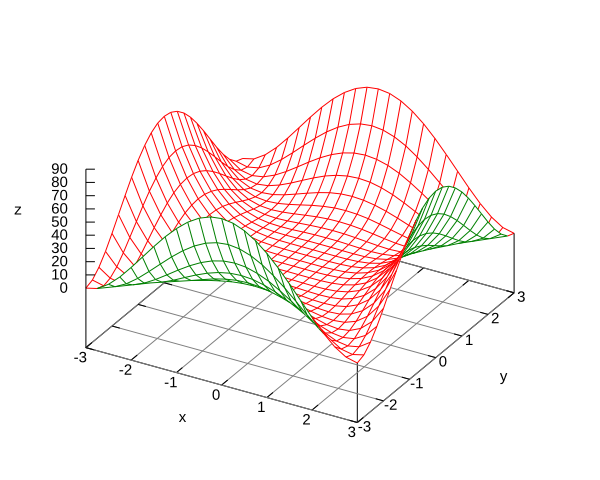c, e, a, b, f, d
2. Use traces to sketch the surface $y={z}^{2}-{x}^{2}$.
1. What does the equation ${x}^{2}+{y}^{2}=1$ represent in ${ℝ}^{2}$?
2. What does it represent in ${ℝ}^{3}$?
3. What does the equation ${x}^{2}+{z}^{2}=1$ represent in ${ℝ}^{3}$?
1. A circle of radius $1$ centered at the origin.
2. A circular cylinder of radius $1$ with axis the $z\text{-axis}$.
3. A circular cylinder of radius $1$ with axis the $y\text{-axis}$.